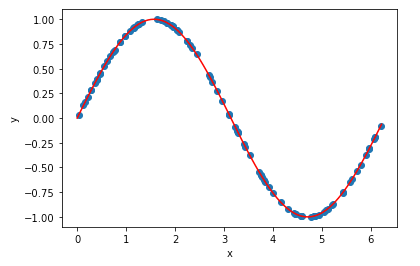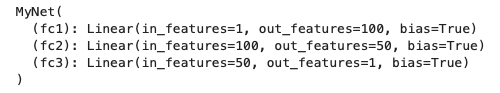# How to use TensorBoard in an Amazon SageMaker Notebook InstanceIn this article, I will show you how to use TensorBoard in an amazon sagemaker notebook instance.

In this article, I will show you how to use TensorBoard in an amazon sagemaker notebook instance.

In one of my recent projects, I needed to visualize training and validation loss using TensorBoard in a sagemaker notebook instance. However, after searching online, I realized that there is rare blog covering this topic. So I wrote this step-by-step tutorial and hopefully it can help you.

Let’s dive into this topic using a simple regression example.

## 1. Create sample data

Firstly, I’m going to create a sample data set using NumPy and simply will create a variable called x, which is a sample of random data from 0 to 2π. Then we will compute y, which is the sign of x, where x is a random data. So x is our input values and y will be our truth for our dataset.

``````import numpy as np
import matplotlib.pyplot as plt

np.random.seed(42)
x = 2 * np.pi * np.random.random((100,1))
y = np.sin(x)

plt.plot(x, y,'o')
plt.xlabel('x')
plt.ylabel('y')

x_all = np.arange(0,2*np.pi, 2*np.pi/100).reshape(-1,1)
plt.plot(x_all, np.sin(x_all), 'r')``````

We can go ahead and plot that out. the blue dots represent our sample dataset. And the red line illustrate what the sign of x would be.## 2. Build neural network

Then let’s build a simple fully connected neural network. This is a very simple neural network. It has two hidden layers. We’re going to use 100 nodes in the first hidden layer and 50 nodes in the second hidden layer. And use the ReLu activation for each of these layers.

``````import torch.nn as
import torch.nn.functional as F

class MyNet(nn.Module):

def __init__(self):
super(MyNet, self).__init__()
self.fc1 = nn.Linear(1,100)
self.fc2 = nn.Linear(100,50)
self.fc3 = nn.Linear(50,1)

def forward(self, x):
x = F.relu(self.fc1(x))
x = F.relu(self.fc2(x))
return self.fc3(x)

model = MyNet()
print(model)``````

If we print the model, we can see a print out of what the model looks like.This doesn’t show us a lot of information visually, but it gives us the information of how many nodes are in each layer and how the layers are connected.

## Using TensorBoard in an Amazon SageMaker PyTorch Training job

Understanding the data flow of an Amazon SageMaker training job, and learning how to set up a TensorBoard on a SageMaker Pytorch training job by examples.

## Hire AWS Developer

Looking to Hire Professional AWS Developers? The technology inventions have demanded all businesses to use and manage cloud-based computing services and Amazon is dominating the cloud computing services provider in the world. **[Hire AWS...

## AWS Cost Allocation Tags and Cost Reduction

Learn more about AWS Cost Allocation Tags, tag examples via the web, CLI, and node.js, explore tag compliance services, and review tagging strategies.

## Single line distributed PyTorch training on AWS SageMaker

How to iterating faster on your data science project, and let your brilliant idea to see the light of day. In this post, I’d like to show you how easy (and cheap, if you want) it is to distribute existing distribution-ready PyTorch training code on AWS SageMaker using simple-sagemaker.

## Deploy your own model with AWS Sagemaker

Sagemaker is a fully managed machine learning service,which provides you support to build models using built-in-algorithms, with native support for bring-your-own-algorithms and ML frameworks such as Apache MXNet, PyTorch, SparkML, Tensorflow and Scikit-Learn.